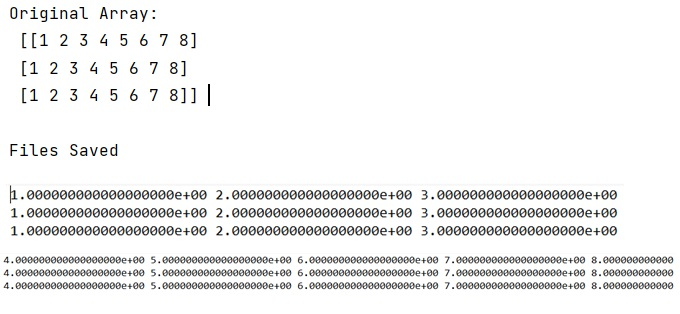# How to write a multidimensional array to a text file?

Learn, how to write a multidimensional array to a text file?
Submitted by Pranit Sharma, on December 26, 2022

NumPy is an abbreviated form of Numerical Python. It is used for different types of scientific operations in python. Numpy is a vast library in python which is used for almost every kind of scientific or mathematical operation. It is itself an array which is a collection of various methods and functions for processing the arrays.

## Writing a multidimensional array to a text file

A multi-dimensional array can be considered as an array that contains multiple rows and columns.

Suppose we are given a multi-dimensional array and we need to look for an efficient way to write this array into a text file.

If we want to write the data into a disk so that its retrieval is easy as a NumPy array, we can use numpy.save() method. If we want to save it into a human-readable format, we need to use numpy.savetxt().

The numpy.savetxt() chokes on ndarrays with more than 2 dimensions. This is probably by design, as there is no inherently defined way to indicate additional dimensions in a text file.

Let us understand with the help of an example,

## Python code to write a multidimensional array to a text file

```# Import numpy
import numpy as np

# Creating a numpy array
arr = np.array([[1,2,3,4,5,6,7,8],[1,2,3,4,5,6,7,8],[1,2,3,4,5,6,7,8]])

# Display original array
print("Original Array:\n",arr,"\n")

# Saving the numpy array
np.savetxt('arr.txt', arr)

# Display a message
print("Files Saved")
```

Output: